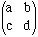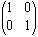Subject: Matrices Information I am an OAC student at Governor Simcoe Secondary School in St. Catharines Ontario. Today at school we were handed an independent study project by our Finite teacher. This assignment is based on Matrices, it involves performing some simple matrix calculations, but also involves a written section. This written section asks for the following: Give a mathematical definition of an identity matrix   Use matrix operations (addition, subtraction,multiplication, scalar multiplication, etc.) to explore the properties of the identity matrix. Including calculations as well as a concise summary of your findings.   Explain and briefly discuss three practical applications of matrices. William Avery Hi William, An identity matrix has to be square and I would describe it by saying that the elements on the main diagonal are 1 while the remainder of the elements are 0. This however is not a mathematical definition. To give a mathematical definition you need to use subscripts. Suppose that A is an n by n matrix with the element in the ith row and jth column written ai,j then A is an identity matrix if ai,j = 1 if i = j, and ai,j = o if i ¬= j where ¬= means "not equal to"  I would start by looking at 2 by 2 matrices. Take the matrices A =and I =and calculate A + I, A - I, A · I, I + A,...   Go to the Quandaries and Queries section of Math Central, search in the keyword field for the word matrix and read "Taxis in Chicago". If you then go to the Resource Room and search for matrix there is description of using matrices to solve systems of equations. Cheers, Harley Go to Math Central